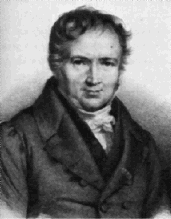A# Poisson, Siméon Denis (1781–1840)

Siméon Poisson was a French mathematician and scientist whose main interest lay in the application of mathematics to physics, especially in electrostatics and magnetism. He developed a two-fluid theory of electricity and provided theoretical support for the experimental results of others, notably Charles de Coulomb. Poisson also made important contributions to mechanics, especially the theory of elasticity; to optics; to the calculus, especially definite integrals; to differential geometry; and to probability theory.

Poisson extended the work of Joseph Louis Lagrange on celestial mechanics and worked on integration and the Fourier series. In Researches on the Probability of Opinions (1837), he presented the Poisson distribution, which gives the probability of the occurrence of a specific event among a large number of events. Poisson's ratio, which relates lateral contraction to longitudinal extension in stressed materials, is also named after him. In all he wrote more than 300 papers on mathematics, physics, and astronomy, and his Traité de mécanique (1811) was long a standard work.